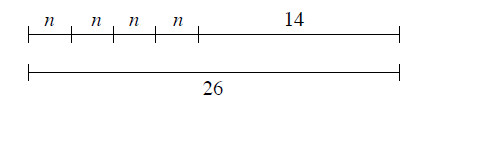### Home > MC1 > Chapter 8 > Lesson 8.3.2 > Problem8-95

8-95.

Write an equation that represents the diagram below and find the value of $n$.Refer to problem 8-94 for help solving an equation for a variable.

You can use $n + n + n + n = 12$.
Why does this equation make sense?